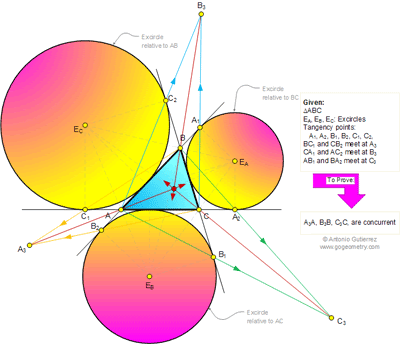## Friday, November 18, 2011

### Problem 689: Triangle, Three Excircles, Tangency points, Tangent lines, Concurrent Lines, Mind Map

Geometry Problem
Level: Mathematics Education, High School, Honors Geometry, College.

Click the figure below to see the complete problem 689.#### 1 comment:

1.Let A₃A∩BC=X, B₃B∩AC=Y, C₃C∩AB=Z
Y,A₁,C₂, lie respectively on the sides CA, AB, BC
of ∆ABC such that YB, CA₁,AC₂ concur at B₃.
∴By Ceva’s Theorem,
(AY/YC)(CC₂/C₂B)(BA₁/A₁A) = 1
∴ AY/YC = (C₂B/CC₂).(A₁A/BA₁)
=[(s-a)/s].[s/(s-c)]
= (s-a)/(s-c)
Similarly,
BZ/ZA = (s-b)/(s-a) and
CX/XB = (s-c)/(s-b)
∴ (AY/YC).(BZ/ZA).(CX/XB)=1
Hence by Converse of Ceva’s Theorem,
AA₃(X), BB₃(Y), CC₃(Z) are concurrent